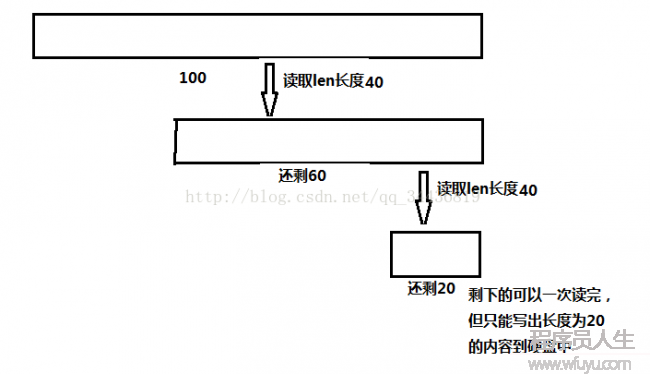# 文件分割器的实现```package com.tiantang.split;

import java.io.BufferedOutputStream;
import java.io.File;
import java.io.FileOutputStream;
import java.io.IOException;
import java.io.OutputStream;
import java.io.RandomAccessFile;
import java.util.ArrayList;
import java.util.List;

public class SplitFile {

//被分割文件的路径
private String srcPath;
//被分割的文件对象
private File src;
//分割的块数
private int size;
//每块的大小
private long blockSize;
//每块的实际大小
private long actualBlockSize;
//原文件的长度
private long length;
//分割得到的文件名的集合
private List<String> splitFileNames=new ArrayList<String>();

public SplitFile(String srcPath,long blockSize){
this.srcPath=srcPath;
this.blockSize=blockSize;
init();
}

/**
* 初始化文件分隔需要用到的参数
*/
private void init(){
//路径为空，则抛出异常
if(srcPath==null){
throw new RuntimeException("文件路径不合法");
}

src=new File(srcPath);

//文件是不是存在
if(!src.exists()){
throw new RuntimeException("文件不存在");
}
//是不是是文件夹
if(src.isDirectory()){
throw new RuntimeException("不能分割文件夹");
}

//如果文件存在
length=src.length();

if(length<this.blockSize){
this.blockSize=length;
}

//计算分割的块数
size=(int) ((length⑴)/this.blockSize+1);
//初始化分割后的文件名集合
initSplitFileNames();
}

/**
* 初始化文件被分割后新生成文件的名字
* 格式为：原文件名+第几块+扩大名
*/
private void initSplitFileNames() {
//文件的全名（包括后缀）
String fileName=src.getName();
//取得文件的扩大名前的分隔符‘.’
int index=fileName.indexOf('.');
//文件名的前缀
String prefixName=fileName.substring(0,index );
//文件名的后缀
String extName=fileName.substring(index);
for(int i=0;i<size;i++){
}
}

/**
* 文件分割的详细细节
*/
public void split(){
RandomAccessFile raf=null;
OutputStream os=null;
try {
raf=new RandomAccessFile(src,"r");
byte[] b=new byte;
int len=0;
for(int i=0;i<size;i++){
raf.seek(blockSize*i);

//计算最后1块的实际大小
if(i==(size⑴)){
actualBlockSize=length-blockSize*(size⑴);
}else{
actualBlockSize=blockSize;
}

os=new BufferedOutputStream(new FileOutputStream(new File(src.getParent(),splitFileNames.get(i))));
//如果读取的长度已超过了实际的长度，则只需要写实际长度的数据
if(len>=actualBlockSize){
os.write(b, 0, (int) actualBlockSize);
os.flush();
break;
}else{
os.write(b, 0, len);
os.flush();
actualBlockSize-=len;
}
}
}
} catch (Exception e) {
e.printStackTrace();
}finally{
if(os!=null){
try {
os.close();
} catch (IOException e) {
e.printStackTrace();
}
}
if(raf!=null){
try {
raf.close();
} catch (IOException e) {
e.printStackTrace();
}
}

}
}

//测试
public static void main(String[] args) {
SplitFile sf=new SplitFile("F:\\test\\tiantang\\java1\\TestException.java",200);
sf.split();
}

}
```------分隔线----------------------------

------分隔线----------------------------3粉丝
0关注